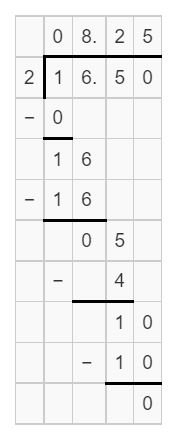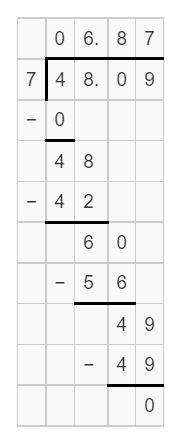# Math in Focus Grade 6 Chapter 14 Answer Key Measures of Central Tendency

This handy Math in Focus Grade 6 Workbook Answer Key Chapter 14 Measures of Central Tendency detailed solutions for the textbook questions.

## Math in Focus Grade 6 Course 1 B Chapter 14 Answer Key Measures of Central Tendency

### Math in Focus Grade 6 Chapter 14 Quick Check Answer Key

Divide.

Question 1.
16.5 ÷ 2
8.25
Explanation:
The given expression is:
16.5 ÷ 2
We know that,
The number before “÷” is called a “Dividend”
The number after “÷” is called a “Divisor”
Now, by using the Long Division,Hence, from the above,
We can conclude that the value of 16.5 ÷ 2 is: 8.25

Question 2.
48.09 ÷ 7
6.87
Explanation:
The given expression is:
48.09 ÷ 7
We know that,
The number before “÷” is called a “Dividend”
The number after “÷” is called a “Divisor”
Now, by using the Long Division,Hence, from the above,
We can conclude that the value of 48.09 ÷ 7 is: 6.87

Find the average of each data set.

Question 3.
2, 4, 16, 18
10
Explanation:
The given data set is:
2, 4, 16, 18
We know that,
Average = $$\frac{The sum of the given data set}{The number of data present in the data set}$$
So, Average = $$\frac{2 + 4 + 16 + 18}{4}$$
= $$\frac{40}{4}$$
= 10
Hence, from the above,
We can conclude that the average of the given Data set is: 10

Question 4.
3, 5, 7, 8, 21, 31
12.5
Explanation:
The given data set is:
3, 5, 7, 8, 21, 31
We know that,
Average = $$\frac{The sum of the given data set}{The number of data present in the data set}$$
So, Average = $$\frac{3 + 5 + 7 + 8 + 21 + 31}{6}$$
= $$\frac{75}{6}$$
= 12.5
Hence, from the above,
We can conclude that the average of the given Data set is: 12.5

Question 5.
$14,$30, $32,$50
$31.5 Explanation: The given data set is:$14, $30,$32, $50 We know that, Average = $$\frac{The sum of the given data set}{The number of data present in the data set}$$ So, Average = $$\frac{14 + 30 + 32 + 50}{4}$$ = $$\frac{126}{4}$$ =$31.5
Hence, from the above,
We can conclude that the average of the given Data set is: \$31.5

Question 6.
25 ft, 32 ft, 45 ft, 55 ft, 75 ft
Average = $$\frac{The sum of the given data set}{The number of data present in the data set}$$
So, Average = $$\frac{25 ft + 32 ft + 45 ft + 55 ft + 75 ft}{5}$$
= $$\frac{232 ft}{5}$$